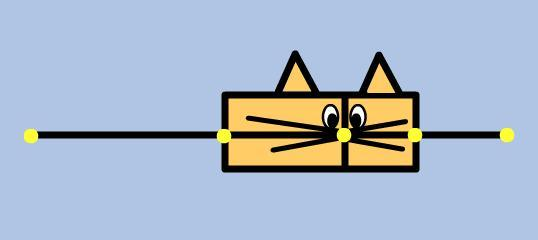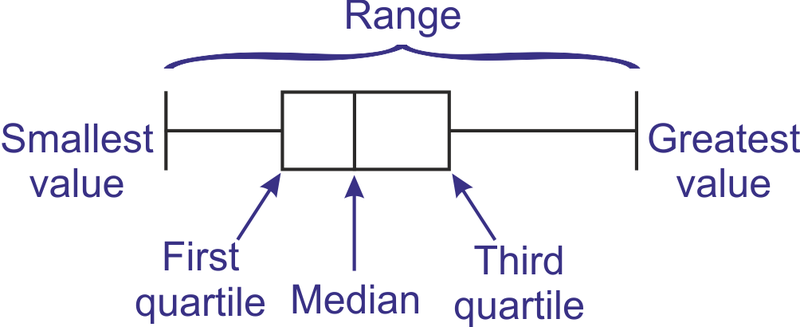# Understanding Box and Whisker Plots as used in the Literacy and Numeracy for Adults Assessment ToolIf you are using the Literacy and Numeracy for Adults Assessment Tool it’s likely that you will have come across the various reporting features available. One of these is the ability to generate a group progress report.

This report is very useful in analysing what is going on with your learners over time. However, the report uses something called a Box and Whisker Plot. And you need to know how the Box and Whisker Plot works if you want to make sense of the data.

What follows below is notes from some work we did today with Department of Corrections staff around how to make sense of this report. It’s part of an embedded numeracy lesson that happens when we teach concepts around diagnostic assessment as part of the NCALNE (Voc) professional development that we deliver.

The following notes come courtesy of Janet Hogan. Many thanks Janet…!

# What’s a Box and Whisker Plot?

A box and whisker plot is a quick way of examining one or more sets of data on a graph. It’s used to measure change, or in the case of the Assessment tool – improvement in assessment scores over time. It’s also part of Statistics which is all about summarising numbers.

The reports generated by the Literacy and Numeracy for Adults Assessment Tool use box and whisker plots to see whether a group of learners have improved their literacy and numeracy between two or more assessments.

A box and whisker plot is based on a five number summary of the data as follows.

• Smallest value or minimum
• First or lower quartile
• Median
• Third or upper quartile
• Greatest value or maximum.The box is drawn around the first and third quartile and the whiskers extend to the smallest and greatest value.

Just a note about the median. This is one of several names for the middle or three different kinds of average if you want to think about it in that way. The two other names of the middle are the mean and the mode. The mean is what we often think about when we talk about the average of a set of numbers. The mode is the number that appears the most.

Ok… with that in mind, let’s have a look at an example of the Group Progress Report from the Assessment Tool.# Example from the Assessment Tool Group Progress Report

In our example there are 22 scores. We can write this as follows:

• n (or the number of scores) = 22.

Don’t be scared off by this way of writing things down. This is the idea of algebra where a letter stands for an idea.

Here are the actual scores, also known as the raw scores. They’re written out in a line from smallest to biggest.

51, 54, 69, 78, 79, 182, 190, 213, 240, 312, 360, 382, 395, 400, 423, 430, 444, 460, 478, 500, 512, 520

• 51 is the smallest score and 520 is the greatest score
• The median is the middle value. One way to find the place of the median score is to add 1 to the number of scores and divide by 2. In our example that would 23 divided by 2 which is 11½. The 11th score is 360 and the 12th score is 382.  Half way between these is 371. The median is 371.
• The first or lower quartile is the middle of the scores below the median. In our example there are 11 scores below the median. Using the same method as above the place of the lower quartile is 12 divided by 2 which is 6. The 6th score is 182.
• The third or upper quartile is the middle of the scores above the median. In our example there are 11 scores above the median. Using the same method as above the place of the upper quartile is 12 divided by 2 which is 6.  The 6th score is 444.

The idea with the 5 number summary and the Box and Whisker Plots is that it allows us to make more informed comments about our whole group and how they are progressing over time. For example, the Box and Whisker Plots make the outliers immediately visible so that these scores aren’t skewing your understanding of how most of the group is progressing.## Author: Graeme Smith

Education, technology, design. Also making cool stuff...